Question

# Hockey puck A is shot along the x-axis at 5.60 m/s at identicalhockey puck B...

Hockey puck A is shot along the x-axis at 5.60 m/s at identical hockey puck B which is initially at rest on horizontal frictionless ice. The collision is off-center, and they scatter at the angles shown.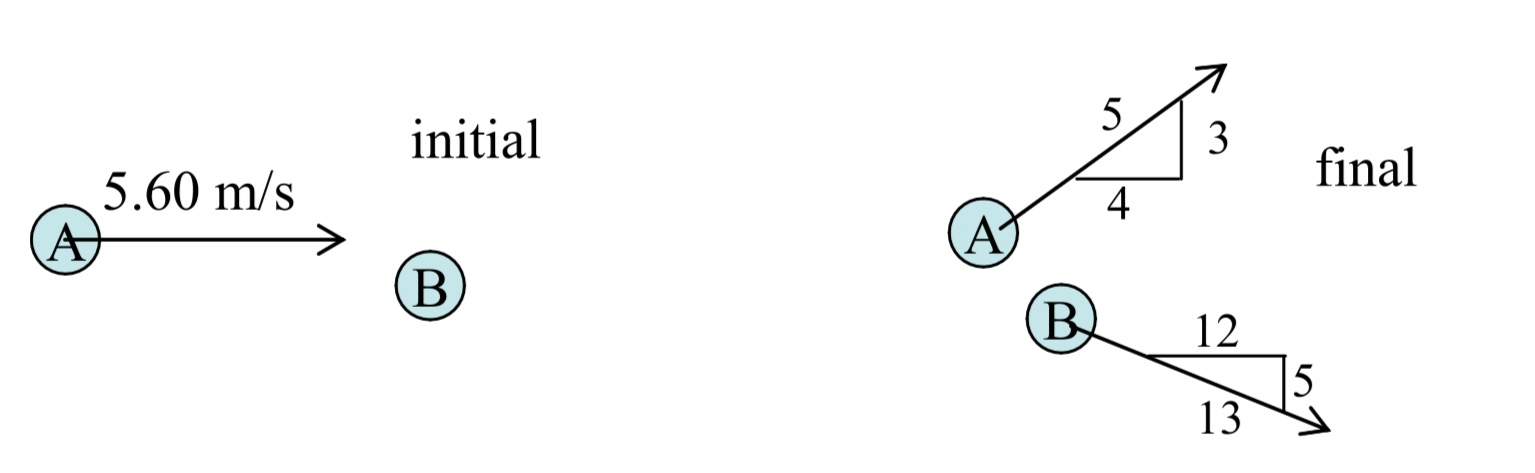a)  Find the speeds vA2 and vB2 of the pucks after the collision.
b)  Find the percentage of kinetic energy converted into other forms during this collision.
c)  Determine the coefficient of restitution for this collision. (Note: Since B is at rest initially, the given final direction of B defines the line of impact and hence normal direction.)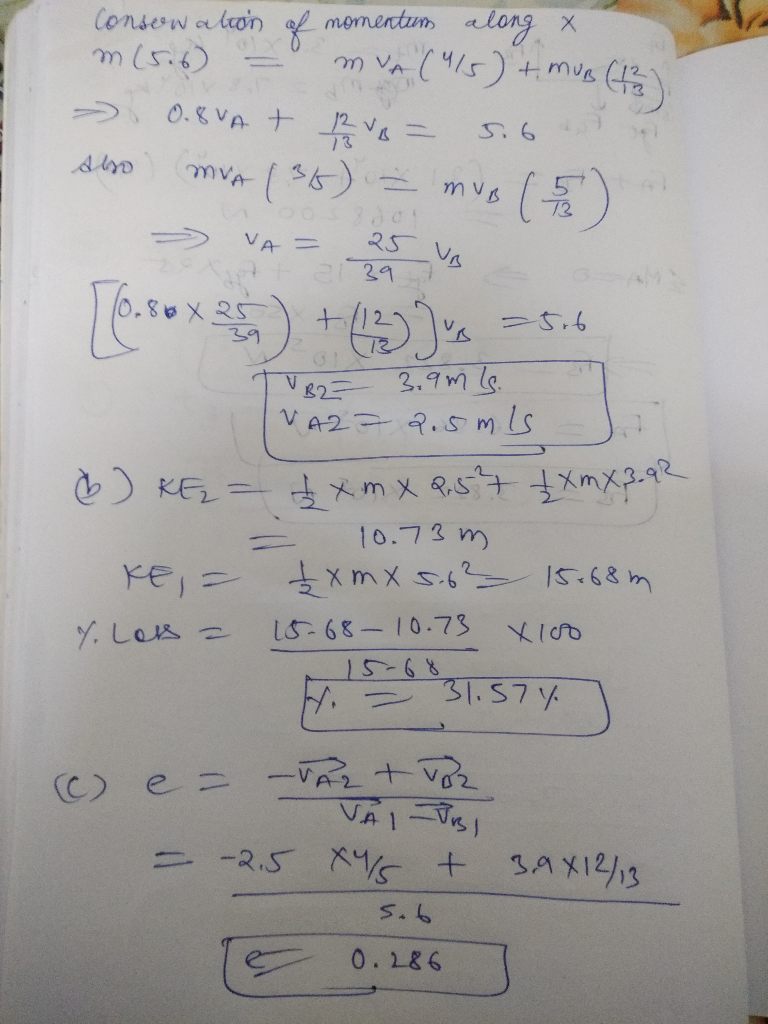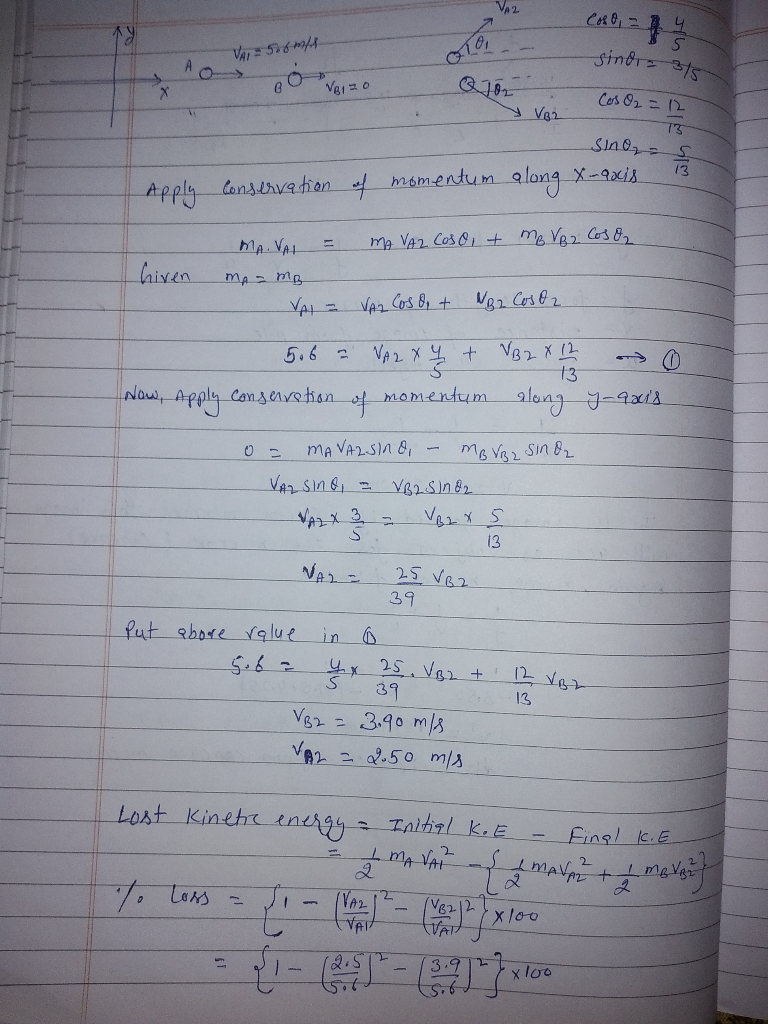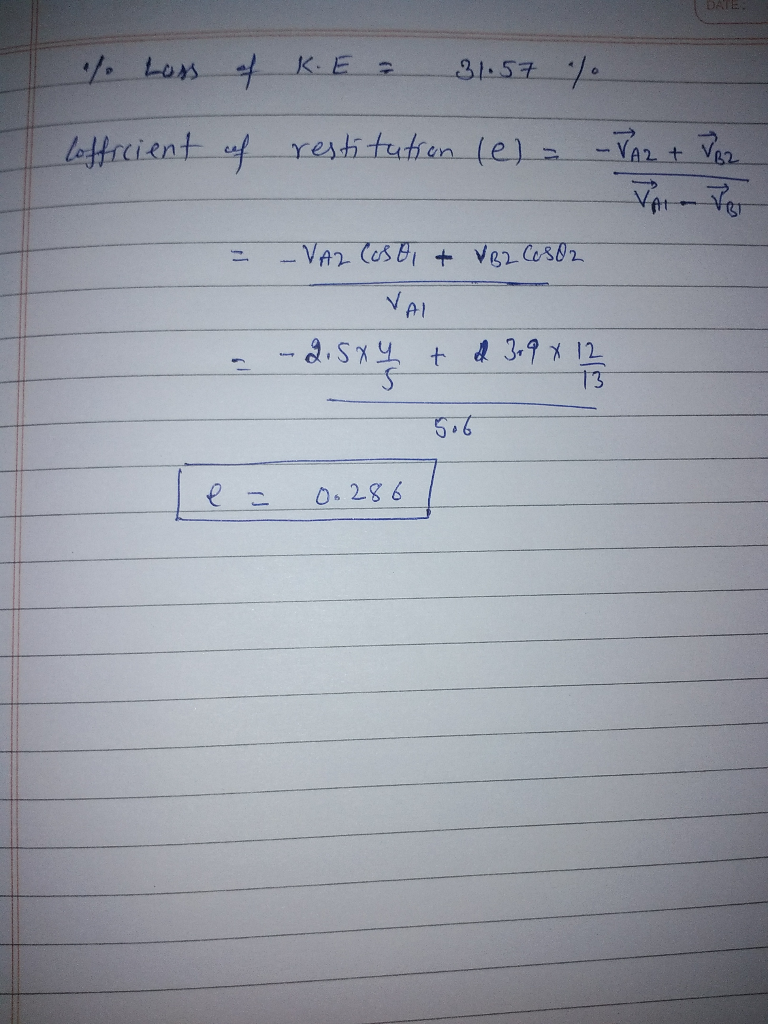#### Earn Coins

Coins can be redeemed for fabulous gifts.

Similar Homework Help Questions
• ### please show all steps and write legibly so i can read. thanks. 6.  (Phys 144...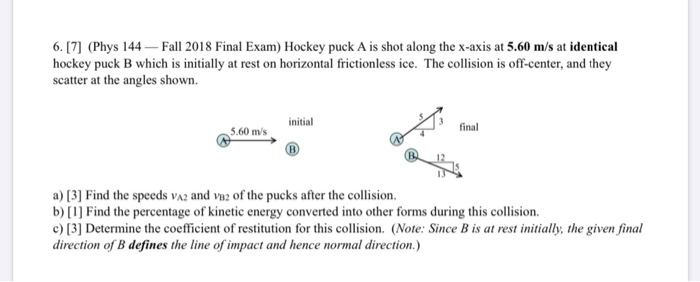please show all steps and write legibly so i can read. thanks. 6.  (Phys 144 Fall 2018 Final Exam) Hockey puck A is shot along the x-axis at 5.60 m/s at identical hockey puck B which is initially at rest on horizontal frictionless ice. The collision is off-center, and they scatter at the angles shown. initial final 5.60 m/s a)  Find the speeds VA2 and vaz of the pucks after the collision. b)  Find the percentage of...

• ### The drawing shows a collision between two pucks on an air-hockey table. Puck A has a...

The drawing shows a collision between two pucks on an air-hockey table. Puck A has a mass of 0.26 kg and is moving along the x axis with a velocity of 5.60 m/s. It makes a collision with puck B, which has a mass of 0.52 kg and is initially at rest. After the collision, the two pucks fly apart with angles as shown in the drawing (α = 56° and β = 40°). Find the final speed of puck...

• ### A hockey puck of mass m = 0.170 kg is loaded into a spring gun with...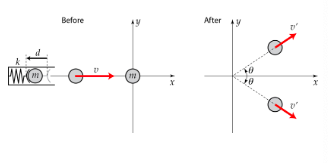A hockey puck of mass m = 0.170 kg is loaded into a spring gun with spring constant k = 306 N/m. The spring is compressed by a distance d = 0.100 m and then released, launching the puck onto a horizontal and frictionless surface of ice with speed v in the positive x-direction. This puck then collides with another puck of the same mass which is at rest at the origin. After the collision the two pucks move away...

• ### A hockey puck B rests on frictionless, level ice and is struck by a second puck A, which was originally traveling at 40.0 m/s and which is deflected 30 degrees from its original direction

A hockey puck B rests on frictionless, level ice and is struck by a second puck A, which was originally traveling at 40.0 m/s and which is deflected 30 degrees from its original direction. Puck B acquires a velocity at a -45 degree angle to the original direction of A. The pucks have the same mass. a) compute the speed of each puck after the collision b) what fraction of the original kinetic energy of puck A dissipates during the...

• ### The drawing shows a collision between two pucks on an air-hockey table. Puck A has a...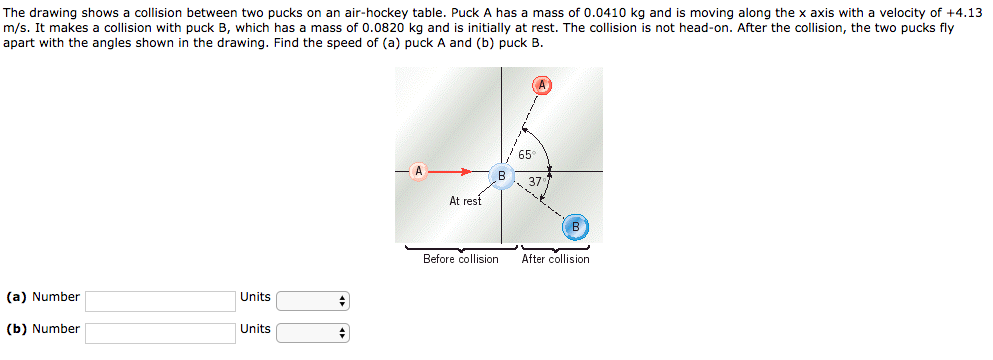The drawing shows a collision between two pucks on an air-hockey table. Puck A has a mass of 0.0410 kg and is moving along the x axis with a velocity of +4.13 m/s. It makes a collision with puck B, which has a mass of 0.0820 kg and is initially at rest. The collision is not head-on. After the collision, the two pucks fly apart with the angles shown in the drawing. Find the speed of (a) puck A and...

• ### The drawing shows a collision between two pucks on an air-hockey table. Puck A has a...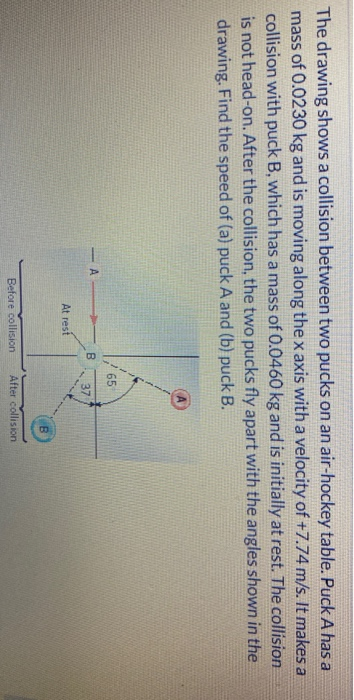The drawing shows a collision between two pucks on an air-hockey table. Puck A has a mass of 0.0230 kg and is moving along the x axis with a velocity of +7.74 m/s. It makes a collision with puck B, which has a mass of 0.0460 kg and is initially at rest. The collision is not head-on. After the collision, the two pucks fly apart with the angles shown in the drawing. Find the speed of (a) puck A and...

• ### A hockey puck of mass 0.39 kg is shot west at 2.90 m/s strikes a second...

A hockey puck of mass 0.39 kg is shot west at 2.90 m/s strikes a second puck, initially at rest, of mass 0.55 kg. As a result of the collision, the first puck is deflected at an angle of 33° south of west, with a speed of 1.45 m/s. What is the speed of the second puck after the collision?

• ### Two pucks collide on an air hockey table. Puck A has a mass of 17.0 g...

Two pucks collide on an air hockey table. Puck A has a mass of 17.0 g and is initially traveling in the +x direction at 7.80 m/s. Puck B has a mass of 68.0 g and is initially at rest. After the pucks collide, puck A moves away at an angle of 58.0 above the +x axis, while puck B travels at an angle of 24.0 below the +x axis. Calculate puck A's final speed? Calculate puck B's final speed?...

• ### 6. The drawing shows a collision between two pucks on an air-hockey table. Puck A has...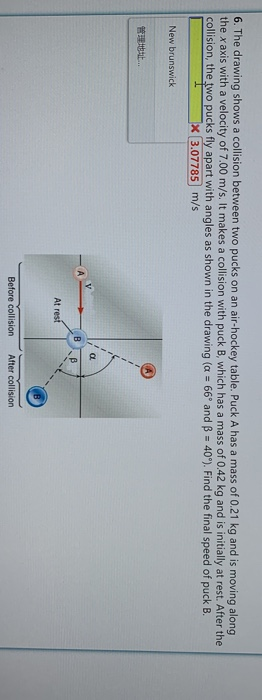6. The drawing shows a collision between two pucks on an air-hockey table. Puck A has a mass of 0.21 kg and is moving along the x axis with a velocity of 7.00 m/s. It makes a collision with puck B, which has a mass of 0.42 kg and is initially at rest. After the collision, the two pucks fly apart with angles as shown in the drawing (a 66° and B 40°). Find the final speed of puck B....